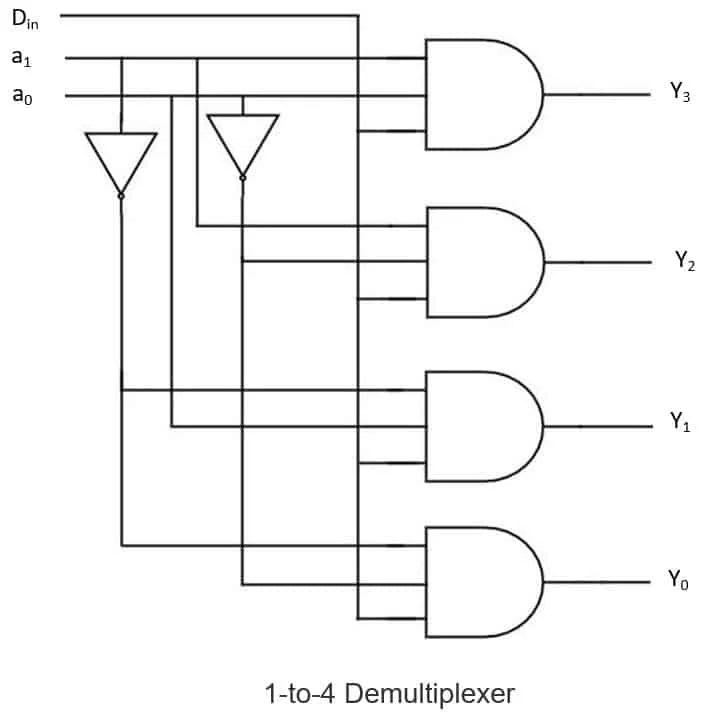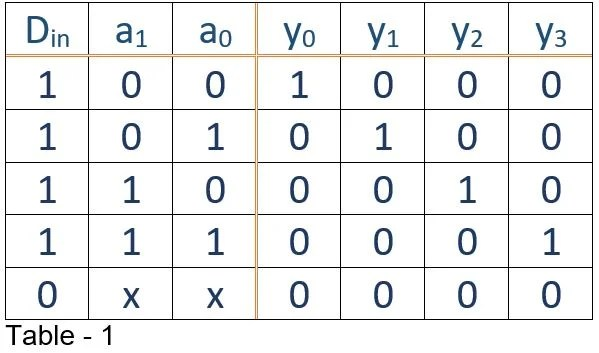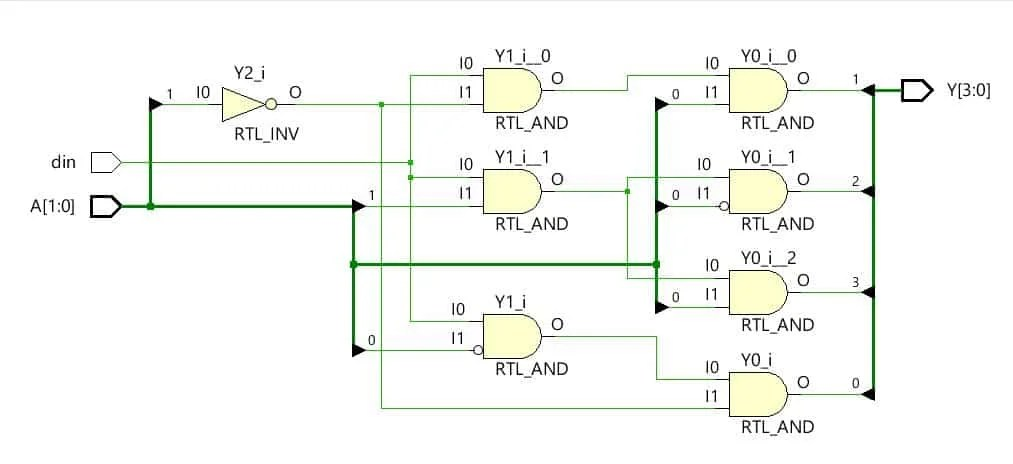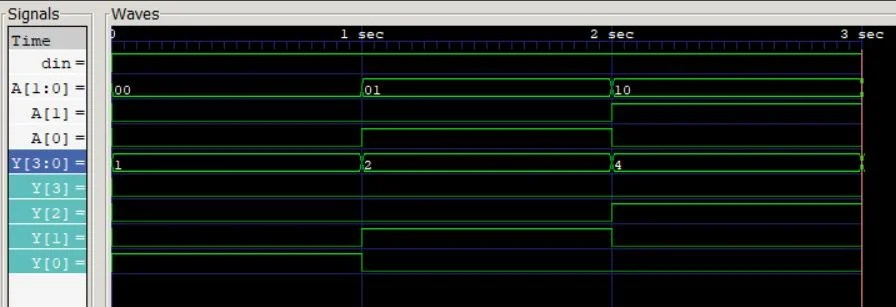View Course Path

# Verilog Code for Demultiplexer Using Behavioral Modeling

Contents

## What is a demultiplexer?

A demultiplexer is a circuit that places the value of a single data input onto multiple data outputs. The demultiplexer circuit can also be implemented using a decoder circuit.

Here we are going to work with 1-to-4 demultiplexer. A 1-to-4 demultiplexer consists of

• one input data line,
• four outputs, and
• two control lines to make selections.

The below diagram shows the circuit of the 1-to-4 demultiplexer. Here a1 and a0 are control or select lines y0, y1, y2, y3 are outputs, and Din is the data line.Now, let’s observe its truth table –The values of a1a0 determine which of the outputs are set to the value of Din. When Din=0, all the outputs are set to 0, including the one selected by the valuation of a1a0. When Din=1, the valuation of a1a0 sets the appropriate output (anyone from y0, y1, y2, y3) to 1.

Now that we have thoroughly understood the concepts of the demultiplexer, let’s dive directly into the Verilog code for the demultiplexer.

## Different methods used in behavioral modeling of a demultiplexer

There are various styles of writing Verilog code in behavioral modeling for this circuit.

1. case statements
2. assignment statements
3. if-else statements

Here we will be elaborating on the first two. Along the way, we would also emphasize some common design errors.

## Verilog code for demultiplexer – Using case statements

The basic building block in Verilog HDL is a module, analogous to the ‘function’ in C. The module declaration is made as follows:

`module Demultiplexer_1_to_4_case (output reg [3:0] Y, input [1:0] A, input din);`

For starters, `module` is a keyword. It is followed by an identifier. Identifier=name of the module. After naming the module, in a pair of parentheses, we specify:

• the direction of a port as input, output or inout.
• Port size, and
• port name.

Taking into consideration the first line of the code, `Demultiplexer_1_to_4_case` is the identifier, the `input` is called port direction. If a port has multiple bits, then it is known as a vector. Hence, `[1:0]` states that the port named as  `A` is a vector with MSB = 1 and LSB = 0.

The `reg` data object holds its value from one procedural assignment statement to the next and means it holds its value over simulation data cycles.

Another style of declaration in the port list is to declare the port size and port direction after the module declaration.

```module Demultiplexer_1_to_4_case (Y, A, din);
output reg [3:0] Y;
input [1:0] A;
input din;
```

`[3:0]` here signifies that the output is of 4 bits. Next up, since its behavioral modeling style, here comes the `always` statement.

```always @(Y, A) begin
```

Using the `always` statement, a procedural statement in Verilog, we will run the program sequentially. `(Y, A)` is known as the sensitivity list or the trigger list. The sensitivity list includes all input signals used by the `always` block. It controls when the statements in the always block are to be evaluated. `@` is a part of the syntax, used before the sensitivity list. In Verilog, `begin` embarks and  `end` concludes any block which contains more than one statement in it.

Note that the always statement `always @(Y, A)` could be written as `always @ *.` ` *` would mean that the code itself has to decide on the input signals of the sensitivity list.

Then inside always block we write,

`case (A)`

The case statement in Verilog is analogous to switch-case in C language. First, let us see the general format to write a case statement in Verilog.

```case (<expression>)
case_item1 :   <single statement>
case_item2 :   <single statement>
case_item3 :   begin
<multiple statements>
end
default    :   <statement>
endcase
```

If the `expression` corresponds to any of the `case_item`, then those design statements are executed. Otherwise, the default case is executed. So, now we can write

```case (A)
2'b00 : begin
Y = din; Y[3:1] = 0;
end
2'b01 : begin
Y = din; Y   = 0;
end
2'b10 : begin
Y = din; Y[1:0] = 0;
end
2'b11 : begin
Y = din; Y[2:0] = 0;
end
endcase
```

As we see here in the first case, `2'b00` represents the case when the input A is `2'b00`. These cases indicate that, according to the value of `A`, one of the four statements is selected. The colon then marks the end of a case item and starts the action that must happen in that particular case. The terms `begin` and `end` are part of the Verilog syntax if you are writing more than one statement in that block. After this, those statements are mentioned, such as the output port `Y` should be attached to the `din, Y[3:0] to 0`, and so on, according to the truth table.

This modeling is based on the behavior of the circuit; hence it is called behavioral modeling. Observe that we are not specifying the structure of the circuit, we are only creating the logic of the circuit which can implement that hardware.

Here is the full code:

```module Demultiplexer_1_to_4_case (output reg [3:0] Y, input [1:0] A, input din);
always @(Y, A) begin
case (A)
2'b00 : begin Y = din; Y[3:1] = 0; end
2'b01 : begin Y = din; Y = 0;   end
2'b10 : begin Y = din; Y[1:0] = 0; end
2'b11 : begin Y = din; Y[2:0] = 0; end
endcase
end
endmodule```

### Test bench for the demultiplexer

The test bench is the file through which we give inputs and observe the outputs. It is a setup to test our Verilog code.

The following line includes the pre-written file Demultiplexer_1_to_4_case.v into the testbench. We start by writing `'include` which is a keyword to include a file. It is followed by the file name in inverted commas.

``include "Demultiplexer_1_to_4_case.v"`

The next line declares the name of the module for testbench according to the syntax as mentioned above. But we do not specify any ports in this module as there will be ports inside the testbench and not outside. Here we declare the data types of the arguments used in the instantiation of the demultiplexer design. A continuous assignment statement assigns values to the `wire` datatype and makes a connection to an actual wire in the circuit.

```module Demultiplexer_1_to_4_case_tb;
```
```wire [3:0] Y;
reg [1:0] A;
reg din;
```

Notice that the inputs in the demux here become the `reg` datatypes and the outputs are specified as `wire`.

Now, we instantiate a module in Verilog.  Instantiation is a very exciting concept and you will be using it frequently in all the examples in this Verilog course.

`Demultiplexer_1_to_4_case Instant0 (Y, A, din);                // syntax for instantiation.`

As in the include file Demultiplexer_1_to_4_case.v  we have a module named `Demultiplexer_1_to_4_case ` which contains our circuit design. Now to test it, we should use the circuit, apply a few inputs, and check the outputs, right? For this purpose, we create an instance.

What happens in Verilog when you create an instance of the circuit?

What actually happens is that the whole circuitry/design gets copied in that testbench or that module, in which it was instantiated. There is nothing like calling of a function (which happens in other programming languages, like C programming) because the code here we are writing is for hardware.

Hardware can not be something where instruction is passed. It has to be there physically, so a copy of that circuit is created in the module where it was instantiated. Now, we write:

```initial begin
din = 1;
A = 2'b00;
#1  A = 2'b01;
#1  A = 2'b10;
#1  A = 2'b11;
end
```

The inputs to the Verilog model are given test values in the initial block. Like `din` is given 1 value, `A` is first given `2'b00`, `2` is the number of bits,`'`(called as a tick), `b` for binary, and the two bits to carry information. `#1` gives a delay of one unit of time in between the test cases. Next is,

```initial begin
\$monitor("%t| Din = %d| A = %d| A = %d| Y = %d| Y = %d| Y = %d| Y = %d",
\$time, din, A, A, Y, Y, Y, Y);
end
```

To view our results in the console, we have the ‘monitor’ keyword. This is housed in an initial block. Note that `%t` is the format specifier for time, `%b` is for binary, `%d` for decimal. The port names after inverted commas are given the same order as required while assigning values. That is all required to build a testbench.

Here is the complete testbench for Verilog code using case statements.

```include "Demultiplexer_1_to_4_case.v"
module Demultiplexer_1_to_4_case_tb;
wire [3:0] Y;
reg [1:0] A;
reg din;
Demultiplexer_1_to_4_case I0 (Y, A, din);
initial begin
din = 1;
A = 2'b00;
#1  A = 2'b01;
#1  A = 2'b10;
#1  A = 2'b11;
end
initial begin
\$monitor("%t| Din = %d| A = %d| A = %d| Y = %d| Y = %d| Y = %d| Y = %d",
\$time, din, A, A, Y, Y, Y, Y);
end
endmodule```

## Verilog code for demultiplexer – Using assignment statement

First of all, we initiate by module and port declaration following the same syntax. We assign identifier as `Demultiplexer_1_to_4_assign`, input as `A`, `din` and output as `Y`.

`module Demultiplexer_1_to_4_assign(output [3:0] Y, input [1:0] A, input din);`

We also set up the size and type of the port, which can only be either input, outputs, or inout.

Then we assign the output as the logical and operation of the select lines and data line. `assign` is a keyword in which the expression or the signal on the right-hand side is evaluated and assigned to the expression on the left side.

```assign Y = din & (~A) & (~A);
assign Y = din & (~A) & A;
assign Y = din & A & (~A);
assign Y = din & A & A;
```

`A` means that we are addressing the zeroth bit of the multi-bit bus, similar goes for `Y`, we are accessing the first bit of Y vector. `&` stands for and operation, `~` is for not operation.

We give all the possible conditions as per our truth table of the demultiplexer.

This is also behavioral modeling as we are not identifying the circuitry, we are only assigning the outputs to bitwise and of data and select lines.

`endmodule`

This marks the end of the module. You may view the complete code here.

```module Demultiplexer_1_to_4_assign(output [3:0] Y, input [1:0] A, input din);
assign Y = din & (~A) & (~A);
assign Y = din & (~A) & A;
assign Y = din & A & (~A);
assign Y = din & A & A;
endmodule```

### Test bench for the demultiplexer

The first line is:

` `include "Demultiplexer_1_to_4_assign.v"`

It includes the Verilog file for the design. Notice that the file name has to be in inverted commas and no semicolon at the end.

Then write:

`module Demultiplexer_1_to_4_assign_tb;`

This assigns an identifier for the testbench and ends in a semicolon. Again, like previous testbench, no ports for the test bench.

```wire [3:0] Y;
reg [1:0] A;
reg din;```

Using keywords such as wire, reg, etc. we define the data type of the ports.

`Demultiplexer_1_to_4_assign Instant1 (Y, A, din);`

The preceding line instantiates the module `Demultiplexer_1_to_4_assign` as `Instant1`, which is an identifier and, the ports are given in the precise order. Then comes the initial block:

```initial begin
din = 1;
A = 0; A = 0;
#1 A = 0; A = 1;
#1 A = 1; A = 0;
#1 A = 1; A = 1;
end```

The initial block contains the test inputs for the design. In the test cases here, we provide din = 1 with all the combinations of A with delays.

Here, this initial block is used to observe the ports and, more importantly, the output ports. `\$time` is the value of the time unit for `%t` format specifier. Other than that, the syntax remains the same.

```initial begin
\$monitor("%t| Din = %d| A = %d| A = %d| Y = %d| Y = %d| Y = %d| Y = %d",
\$time, din, A, A, Y, Y, Y, Y);
end```

`monitor` is a keyword in Verilog, and it displays the contents in the parenthesis on the console. Look at the whole code here.

````include "Demultiplexer_1_to_4_assign.v"
module Demultiplexer_1_to_4_assign_tb;
wire [3:0] Y;
reg [1:0] A;
reg din;
Demultiplexer_1_to_4_assign I0 (Y, A, din);
initial begin
din = 1;
A = 0; A = 0;
#1 A = 0; A = 1;
#1 A = 1; A = 0;
#1 A = 1; A = 1;
end
initial begin
\$monitor("%t| Din = %d| A = %d| A = %d| Y = %d| Y = %d| Y = %d| Y = %d",
\$time, din, A, A, Y, Y, Y, Y);
end endmodule
```

## Hardware schematic for the demultiplexer

Here is the Hardware schematic which you may develop using Xilinx for demultiplexer.## Simulation log for the demultiplexer

Simulation log relating to our truth table.We can observe that din is always 1; all combinations of A are made, the output can be verified easily. For example- A = 0, A = 0, see that the waveform of Y is high.

Thanks for reading! If you have any queries, let us know in the comments section below!

This site uses Akismet to reduce spam. Learn how your comment data is processed.

Top